SELF 74 S.B. Karavashkin and O.N. Karavashkina6.4.2.4. The speed of light radiated by the source falling in the homogeneous gravity field Before we start solving the problem of propagation of light radiated by the uniformly accelerated source from the point of the uniformly accelerated frame, let us pay attention that Einstein also considered this problem in his papers – e.g., [52, section 20], , , – and we in our previous paper [12, p. 2] already analysed the incorrect approaches to its solution made by Einstein in finding the speed of light when in propagates in the gravity field. In this connection we will briefly touch some aspects of Einsteinian solution. As we showed in the subsection 6.4.2.3, in SRT the acceleration of a body or of a frame causes unsolvable difficulties. This made Einstein substituting the exact relationships which are required in this case by those approximate, factually reducing the problem to the approximately classical case, i.e. to small speeds of bodies and frames. But the difficulty also was to retain in these approximations the postulate of constant lightspeed without which the whole formalism of relativistic conception, along with junction of space and time into a single continuum, loses any sense. But Einstein clearly could not yield the constant speed of light in accelerated frames; this brought him to dare to some tricks like this: “Consider first a body whose material points at some moment of time t in a non-accelerated frame S are at rest relative to S but have some acceleration” [52, p. 106]. Or ibidem: “Now consider the frame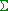uniformly accelerated relative to a non-accelerated frame S in direction with the axis X of this last. Suppose, the coordinate origin of the framemoves along the axis X of the frame S and axes ofare parallel to those of S. At each moment of time there exists a non-accelerated frame S'  whose coordinates of the axis at the considered moment (at the definite moment of time t'  in S' ) coincide with the coordinate axes of frame. If the point event that occurs at the moment of time t'  has inthe coordinates,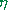,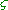, then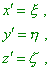(6.51)
 because, according to the said above, we may neglect the affection of acceleration onto the size of body taken to measure,,. Imagine further that the clock inat the moment t'   in S'  go so that the reading at this moment is t'  . Which will be the reading at the next interval of time?” [52, p. 106–107]. Notice that beginning with the moment of time at which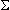and S' had equal speeds, Einstein finishes with the issue of intervals, though it is clear that at the next moment we already have to compare the framewith other frame, S'' , and according just to the Einsteinian principle of non-simultaneity, the time in it at the first moment was not the same as in. And within the relativistic formalism we cannot match these readings in inertial frames, because of inclination of the plane of events. The more we cannot get it over when considering the frames with the changing speed. It looks like Einstein did not much care of it. As we cited above, he same quietly neglects the affection of acceleration onto the pace of time, forgetting that the change of speed automatically has to cause not only the change of pace of time but even worse mismatch of simultaneity of events. We have to mark with it, we may not ignore in general considerations a little value of mismatch, as well as we may not ignore the acceleration, as it makes incorrect our account of the change of speed of frame, i.e. of the statement of problem. None the less, having neglected the effect of acceleration onto the spatial and temporal scales, Einstein concludes: “from this, the light in vacuum propagates relative toduring the element of timewith the universal speed c, if we find the simultaneity in the frame S'  that is resting at the moment relative to, and if we, to measure the time and coordinates, apply respectively clocks and scales equivalent to those which are applied to measure time and space in non-accelerated frames. Thus, in this case also, to define simultaneity, we can apply the principle of lightspeed constancy, if we confine it to very little paths of light” [52, p. 107]. But if we neglected the acceleration in proving the postulates, this does not mean that acceleration fully went away from our consideration. In the next page, considering, how the acceleration affects the local time of frame, Einstein outspokenly writes: “Now find the relationship between the timeand local timeof the point event. From the first equation (1) (the Lorentz transform for inertial frames – Authors) it follows that two events are simultaneous relative to S'  and consequently, relative to(? – Authors), under condition that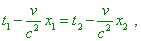(6.52)
 and the indexes indicate to which point event they belong” [52, p. 108]. Let us draw our attention: in the statement of problem Einstein said that the frames S'  andcoincide at one moment of time – i.e. at the moment when the speeds of both frames will be equal. Now he writes the simultaneous events already for two moments of time and at different points of frame S' , and he compares their simultaneity, while, as we already said, at the next moment of time he would have to compare moments already with another frame, and Einstein would never yield his (6.52). Or he would have to ignore the acceleration of the frame, premising that the acceleration does not affect the time processes. But Einstein does not ignore the acceleration and compares namely the pairs of events. He writes: “Let us confine ourselves to considering such time intervals that we could neglect all terms containing the second and higher orders ofor v ; then, given (1) and (29), we have to suppose…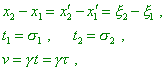(6.53)
 so that for the above relationship we yield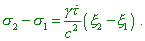(6.54)
 Placing the first point event into the coordinate origin so that1 =and1 = 0  and omitting the index for the second point event, yield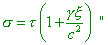(6.55)
 [52, p. 108].  As the result, Einstein yielded a precursor of his known formula that describes the dependence of lightspeed on the gravity potential. With it, if we look at the formulas (6.53), we will see in them the equalised intervals in all three frames – inertial resting S, inertial moving S'  and non-inertial. We also see that according to (6.53), times in S andappear also equal. With it, the speed of framenaturally varies in time, at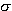1 it will be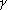1 and at2 it will be2 . An alike level of proof we see when Einstein substantiates interaction of light with gravity field. In the beginning of paper we see the usual for Einstein ascertaining of fact that we have no information about the interaction of light with gravity field: “A priori we can say nothing of the process of transfer of energy through radiation, as we do not know, how the gravity field affects the energy of radiation and the measuring instruments in S1  and S2” [79, p. 168]. Further, and also in a trivial way, Einstein to this indefiniteness which he made introduces the principle of equivalence as the fact that already has been established: “But, according to the admission that frames K and K '  are equivalent, we may, instead the frame K being in the homogeneous field of gravity, put the frame K '  being free of gravity and moving with the uniform acceleration with the positive values of z, while physical frames S1  and S2are strongly connected with this axis z” [79, p. 168] ( see Fig. 1 of the cited Einsteinian work – our Fig. 6.23).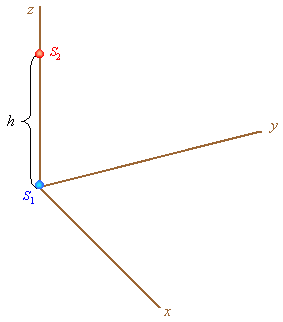Fig. 6.23. Arrangement of physical frames  in the frame K'  that moves with the uniform acceleration [79, p. 168]
 In the previous sections we enough showed, whether it is correct to introduce such equivalence within Relativity, so we will not touch this issue again. We only will pay attention to the fact that in the statement of problem, the physical frames S1 and S2 are connected with the uniformly accelerated frame K'  to which they belong. This will be important for further analysis of Einstein’s proof that the light interacts with the gravity field. Let us continue citing: “Assume, we are discussing the process of energy transfer through radiation from S2  to S1 , while we are located in some non-accelerated frame K0 . Assume, at the moment when the radiation energy E2  is transferred from S2  to S1 , the frame K'   has zero speed relative to K0 . The beams will reach the frame S1  after the time h/c (in the first approximation). At this moment the frame S1  has in K0  the speedh/c = v . So, according to the trivial theory of relativity, the radiation that reaches S1  has not the energy E2  but larger energy E2  that in the first approximation is interrelated with E2  through the relationship ????????????: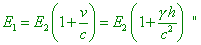(6.56)
 [79, p. 168]. Now let us analyse the cited. According to the statement of problem, we see the propagation of beam from S2 to S1 from the resting frame K0 . According to the trivial theory of relativity, the speed of light propagation is not related to the type of source’s motion. Thus, the lightspeed, as well as the energy transferred by this beam, has to remain constant relative to K0 . So from the point of K0  such change of energy is nonsense. And from the point of K' , in supposition of lightspeed constant in all frames, the energy change due to the change of frame is also nonsense, as from the point of uniformly accelerated frame, the light was radiated by the resting physical frame S2 and was absorbed by also resting physical frame S1 . With it, during the transfer, it in no way was related to S1 or S2 , so it was basically unable to gain energy. The more that we do not consider the very effect of light interacting with gravity field, we do consider kinematics of light propagation in the uniformly accelerated frame, and growth of speed of S1 has no relation to the supposed interaction. Approaching the issue from Einstein’s point, we can state, out of gravity field, the beam of light radiated in some accelerated frame will gain additional energy on the account of linear acceleration of frame, though it has no grounds to do so, if the light propagation is not connected with the state of source’s motion. Just this inconsistency made Einstein to find the energy in one frame from another frame, arbitrarily arranging the parameters of both frames, which is inadmissible both in classical physics and in SRT that uses the formalism of classical physics to describe the motion of body in one frame. Now let us consider, how classical physics will describe the light propagation in the uniformly accelerated frame. To solve, we will broaden the statement of this problem and will seek not the lightspeed in the accelerated frame, as Einstein did – we will graph the equiphase surfaces of radiation of the source moving with the uniform acceleration in the homogeneous gravity field, without a premise that light interacts with this field. Let we have two frames: inertial frame S rests relative to the gravitating centre and the framefreely falling in the quasi-homogeneous field (small region of space) of this centre. The axes x  and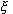of both frames coincide, are directed to the source of field and at the initial moment of time the speed ofis zero. Of course, such conditions assume that the framemoves with the positive values of the axis x of the frame S. Also, let in the uniformly accelerated frame there is a source of light resting in the origin of this frame. From the point of inertial frame, the equations of equiphase surfaces radiated by the source do not differ from the yielded above, in the section 3, equations of moving source (3.8). In case of accelerated source, they will be as follows: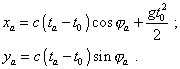(6.57)
 Comparing (6.57) with (3.8), we see the difference only in the second term of the first equation. In (6.57) this summand is quadratically dependent on time, which corresponds to the pattern of source’s motion at the moment when the equiphase surface has been radiated. Now, to yield the equations of equiphase surface in the freely falling frame, we have to use trivial transforms of classical physics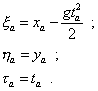(6.58)
 Substituting (6.57) to (6.58), yield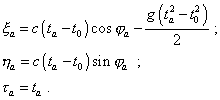(6.59)
 The system (6.59) describes the equiphase surfaces in the freely falling frame. The same or similar solution Einstein had to yield, noting that he neglected the quadratic terms including the speed of freely falling frame and smallness of acceleration of free fall. The dynamic diagram of equiphase surfaces plotted with (6.59) is shown in Fig. 6.24.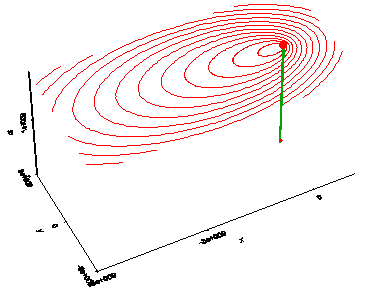Fig. 6.24. Propagation of equiphase surfaces radiated by the uniformly accelerated source from the point of frame in which this source rests
 First of all, we see in this diagram that the density of equiphase surfaces in the wavefront varies in time. This relates to the fact that in the accelerated frame, the speed of light propagation is inconstant in time, and we can show it with the modelling equations (6.59). Actually, we have only to determine the derivative ina :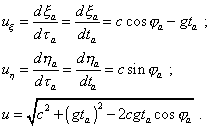(6.60)
 The expressions (6.60) show that in the uniformly accelerated frame, the lightspeed is anisotropic and growing in time, while the source and receiver rest in the accelerated frame. This anisotropy remains at small speeds and small accelerations of free fall of the frame, as under these conditions the last expression in (6.60) will take the appearance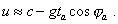(6.61)
 We see that in (6.61) the anisotropy of speed remains, as well as its variation in time. This disables to assume the constant lightspeed in non-inertial frames, neither the equivalence of inertial and non-inertial frames. This non-equivalence is typical not only from the point of classical physics but also from the point of relativistic conception which, as its adherents say, has at little speeds to coincide in its results with the classical theory. It debunks the relativistic statements that “the commonly known physical facts bring us to the general principle of relativity, i.e. to the statement that we have to formulate the laws of nature so that they to be true for arbitrarily moving coordinate systems” [45, p. 423] and ungrounded relativistic accusations that “Newton’s mechanics gives no trustworthy answer to this question. It says the following. The laws of mechanics are true for the space R1  relative to which the body S1  rests but are not true for the space R2  relative to which the body S2  rests. But the Galilean space R1  (and the motion relative to it) that is introduced with it is the fictitious cause, not the observed fact. Thus, it is clear that in the considered case the Newtonian mechanics satisfies the requirement of causation not in its essence but only seemingly, putting the responsibility for the observed different behaviour of bodies S1  and S2  onto the fictitious cause – the space R1 ” [21, p. 455]. As opposite, impossibility to put in agreement both special and general principle of relativity at small speeds of material bodies and, the main, of the sources of field shows the relativistic conception fully incorrect, as well as the relativistic substantiation of equivalence of inertial and non-inertial reference frames is discrepant. The derivation shows, even if we do not use directly the laws of dynamics, we anyway base on them, premising such or other behaviour of the body during and after the interaction with other bodies or fields. Namely from this symbiosis the solution of full value follows. “But now, if in that space some remote bodies were placed the kept always a given position one to another, as the fixed stars do in our regions, we could not indeed determine from the relative translation of the globes among those bodies, whether the motion did belong to the globes or to the bodies. But if we observed the cord, and found that its tension was that very tension which the motions of the globes required, we might conclude the motion to be in the globes, and the bodies to be at rest; and then, lastly, from the translation of the globes among the bodies, we should find the determination of their motions” [2, p. 36]. And if we confine ourselves to kinematics and to an arbitrary interpretation of the laws of dynamics, selecting from them only convenient displays and particular processes, and if we reduce physics to an amount of paradoxes and absurds – to which, basically, Mach’s theory has been reduced by relativists who brought it to the state that terrified the author of revolving vessel paradox. But if speaking of the experience, then, basing on the number of typical calculations that we gave, we can conclude only that just the experience in the diversity of features of models shows the acceleration of material bodies absolute in the meaning that the body’s inertia shows itself only if the body immediately takes a part in the acceleration/deceleration and the very inertia arises as the property of material body, not as the result of interaction with the remote gravitating bodies. “If the body stops, it stops either due to the unequal resistance, when the falling body appears heavier than the medium, as it occurs if we put wood onto wax, or to the equal resistance, as it occurs if we pour water on water either put wood on wood of same kind. This is just to what scholastics give an absolutely idle definition: ‘The body has a weight only out of its place’. This all very differently affects the motion of weight, as the motion of heavy bodies on the weights shows itself otherwise than in the free fall, one thing (though it can seem surprising) is the motion of pans hanged in the air, and another – their motion when we put them to water; one thing is the motion of weight when the body falls in water, and another – when it is on the water surface” [80, p. 315]. In this meaning, we also may not reduce the concept of time only to the fact that the transfer of clocks or their synchronisation through the light signals “is not, as such, the establishment of the observed fact and does not relate to some inner property of clocks either light; this is a simple expression of the rule with which we expect to do the fictitious graduation of time in the whole world” [7, p. 57]. And Einstein wrote it at the time when in the dialogue with Dingle he “underlined the operationalistic approach, according to which the physical concept (e.g., an interval of time) is thought undefined until we did not point the instrumental operation that allows to measure it. His criterion of clocks admittance was based on the interpretation of their reading as the events” [24, p. 152]. It remains only to compare this citation with that Newtonian: “Wherefore relative quantities are not the quantities themselves, whose names they bear, but those sensible measures of them (either accurate or inaccurate), which are commonly used instead of the measured quantities themselves. And if the meaning of words is to be determined by their use, then by the names time, space, place and motion, their measures are properly to be understood; and the expression will be unusual, and purely mathematical, if the measured quantities themselves are meant. Upon which account, they do strain the sacred writings, who there interpret those words for the measured quantities. Nor do those less defile the purity of mathematical and philosophical truths, who confound real quantities themselves with their relations and vulgar measures” [2, p. 35–36].

Contents: / 53 / 54 / 55 / 56 / 57 / 58 / 59 / 60 / 61 / 62 / 63 / 64 / 65 / 66 / 67 / 68 / 69 / 70 / 71 / 72 / 73 / 74 / 75 / 76 / 77 / 78 / 79 / 80 / 81 / 82 / 83 /